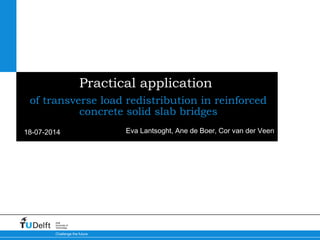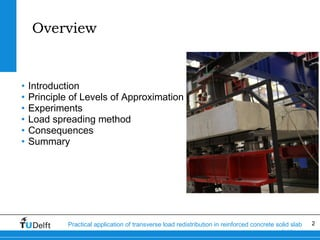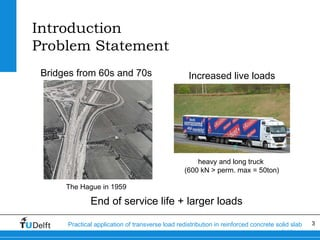Successfully reported this slideshow.

# Practical Application of Transverse Load Redistribution in Reinforced Concrete Solid Slab Bridges×

# Practical Application of Transverse Load Redistribution in Reinforced Concrete Solid Slab Bridges

### Practical Application of Transverse Load Redistribution in Reinforced Concrete Solid Slab Bridges

1. 1. 18-07-2014 Challenge the future Delft University of Technology Practical application of transverse load redistribution in reinforced concrete solid slab bridges Eva Lantsoght, Ane de Boer, Cor van der Veen
2. 2. 2Practical application of transverse load redistribution in reinforced concrete solid slab bridges Overview • Introduction • Principle of Levels of Approximation • Experiments • Load spreading method • Consequences • Summary
3. 3. 3Practical application of transverse load redistribution in reinforced concrete solid slab bridges Introduction Problem Statement Bridges from 60s and 70s The Hague in 1959 Increased live loads heavy and long truck (600 kN > perm. max = 50ton) End of service life + larger loads
4. 4. 4Practical application of transverse load redistribution in reinforced concrete solid slab bridges Introduction Highway network in the Netherlands • NL: 60% of bridges built before 1976 • Assessment: shear critical in 600 slab bridges • LoA I method: Quick Scan Highways in the Netherlands
5. 5. 5Practical application of transverse load redistribution in reinforced concrete solid slab bridges Principle of Levels of Approximation Model Code 2010 • Approach from fib Model Code 2010 • Solution strategy = different levels of approximation • Eg: Shear capacity in Model Code 2010
6. 6. 6Practical application of transverse load redistribution in reinforced concrete solid slab bridges Experiments Size: 5m x 2.5m (variable) x 0.3m = scale 1:2 Continuous support, Line supports Concentrated load: vary a/d and position along width
7. 7. 7Practical application of transverse load redistribution in reinforced concrete solid slab bridges Load spreading Effective width in shear 45° load spreading - Dutch practice 45° load spreading – French practice Or: fixed value (eg. 1m = 3.3ft)
8. 8. 8Practical application of transverse load redistribution in reinforced concrete solid slab bridges Load spreading Results of experiments BS = 0.5m = 1.6 ft wide BX = 2.0m = 6.6ft wide
9. 9. 9Practical application of transverse load redistribution in reinforced concrete solid slab bridges Load spreading Results of experiments 5000 1000 1500 2000 2500 b (mm)
10. 10. 10Practical application of transverse load redistribution in reinforced concrete solid slab bridges Load spreading Statistical analysis • Calculated from series vs. 45° load spreading • Comparison between database (literature) + experiments and methods • French load spreading method underestimates less • Lower COV for French load spreading method • Database: 63% vs 42% • Delft experiments: 26% vs 22%
11. 11. 11Practical application of transverse load redistribution in reinforced concrete solid slab bridges Load spreading Finite element results (1) Models of 1.5m = 4.9ft wide a = center-to-center distance between load and support Effective width from shear stress distribution over support
12. 12. 12Practical application of transverse load redistribution in reinforced concrete solid slab bridges Load spreading Finite element results (2) Models of 2.5m = 8.2ft wide a = center-to-center distance between load and support Effective width from shear stress distribution over support
13. 13. 13Practical application of transverse load redistribution in reinforced concrete solid slab bridges Load spreading Finite element results (3) Models of 3.5m = 11.5ft wide a = center-to-center distance between load and support Effective width from shear stress distribution over support
14. 14. 14Practical application of transverse load redistribution in reinforced concrete solid slab bridges Load spreading Finite element results (4) • French load spreading method gives safe estimate of beff • NLFEA: beff depends slightly on slab width • NLFEA: influence of a/d less than in French method • French method sufficient for LoA 1
15. 15. 15Practical application of transverse load redistribution in reinforced concrete solid slab bridges Load spreading Application to slab bridges (1) • Loading at edge • Asymmetric effective width
16. 16. 16Practical application of transverse load redistribution in reinforced concrete solid slab bridges Load spreading Application to slab bridges (2) Effective width per axle instead of per wheel print
17. 17. 17Practical application of transverse load redistribution in reinforced concrete solid slab bridges Load spreading Consequences • Larger effective width • Smaller shear stress • More economic design • Sharper assessment
18. 18. 18Practical application of transverse load redistribution in reinforced concrete solid slab bridges Summary & Conclusions 1. Level I of Assessment: Quick Scan method 2. French load spreading method 1. Experimental results 2. Statistical analysis 3. Finite element results
19. 19. 19Practical application of transverse load redistribution in reinforced concrete solid slab bridges Contact: Eva Lantsoght E.O.L.Lantsoght@tudelft.nl elantsoght@usfq.edu.ec